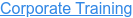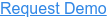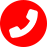# Top 25  Machine Learning Interview Question and Answer

by Shanmugapriya J, on Jun 3, 2023 4:09:30 PM

## 1.What is machine learning?

Ans: Machine learning is a branch of artificial intelligence that focuses on developing algorithms and models that allow computers to learn and make predictions or decisions without being explicitly programmed.

## 2.What are the different types of machine learning?

Ans: The main types of machine learning are supervised learning, unsupervised learning, and reinforcement learning.

## 3.What is supervised learning?

Ans: Supervised learning is a type of machine learning where the model learns from labeled training data, where each data point is associated with a known output or target value. The goal is to learn a function that maps inputs to outputs.

## 4.What is unsupervised learning?

Ans: Unsupervised learning is a type of machine learning where the model learns from unlabeled data, without any specific target variable. The goal is to discover patterns, structures, or relationships in the data.

## 5.What is reinforcement learning?

Ans: Reinforcement learning is a type of machine learning where an agent learns to interact with an environment by receiving feedback in the form of rewards or punishments. The agent learns to take actions that maximize the cumulative reward over time.

## 6.What is the difference between overfitting and underfitting?

Ans: Over                              fitting occurs when a machine learning model performs well on the training data but fails to generalize to new, unseen data. Underfitting, on the other hand, occurs when a model is too simple to capture the underlying patterns in the data, resulting in poor performance on both the training and test data.

## 7.What is the bias-variance tradeoff?

Ans: The bias-variance tradeoff refers to the tradeoff between a model's ability to fit the training data well (low bias) and its ability to generalize to new, unseen data (low variance). Increasing model complexity can reduce bias but increase variance, while decreasing complexity can reduce variance but increase bias.

## 8.What are the steps involved in a typical machine learning project?

Ans: The steps in a typical machine learning project include: a. Data collection and preprocessing b. Exploratory data analysis c. Feature engineering and selection d. Model training and evaluation e. Model deployment and monitoring

## 9.What is cross-validation?

Ans: Cross-validation is a technique used to assess the performance and generalization ability of a machine learning model. It involves partitioning the data into multiple subsets (folds), training the model on a subset, and evaluating it on the remaining fold. This process is repeated multiple times, and the results are averaged.

## 10.What evaluation metrics can be used for classification problems?

Ans: Common evaluation metrics for classification problems include accuracy, precision, recall, F1 score, and area under the ROC curve (AUC-ROC).

## 11.What evaluation metrics can be used for regression problems?

Ans: Common evaluation metrics for regression problems include mean squared error (MSE), root mean squared error (RMSE), mean absolute error (MAE), and R-squared.

## 12.What is the curse of dimensionality?

Ans: The curse of dimensionality refers to the challenges that arise when working with high-dimensional data. As the number of dimensions increases, the data becomes increasingly sparse, and the distance between data points becomes less meaningful. This can lead to difficulties in model training and generalization.

Ans: Ensemble methods combine the predictions of multiple individual models to make a final prediction. The advantages of ensemble methods include improved predictive performance, increased robustness, and the ability to handle complex relationships in the data. However, ensemble methods can be computationally expensive and difficult to interpret.

## 14.What is feature selection and why is it important?

Ans: Feature selection is the process of selecting a subset of relevant features from the original set of features. It is important because it can improve model performance by reducing overfitting, decreasing training time, and improving interpretability.

## 15.What are the main steps in feature selection?

Ans: The main steps in feature selection are: a. Univariate feature selection: Select features based on their individual relationship with the target variable. b. Recursive feature elimination: Iteratively remove less important features based on model performance. c. Model-based feature selection: Use a model to evaluate the importance of each feature. d. Domain knowledge and expert opinion: Consider the relevance of features based on domain knowledge and expert opinion.

## 16.Explain the concept of regularization in machine learning.

Ans: Regularization is a technique used to prevent overfitting in machine learning models. It introduces a penalty term to the model's objective function, which discourages the model from fitting the training data too closely. Common regularization techniques include L1 regularization (Lasso) and L2 regularization (Ridge).

## 17.What is the difference between bagging and boosting?

Ans: Bagging and boosting are both ensemble techniques, but they differ in their approach. Bagging involves training multiple independent models on different subsets of the training data and averaging their predictions. Boosting, on the other hand, involves training models sequentially, where each subsequent model focuses on the errors made by the previous models.

## 18.What is deep learning?

Ans: Deep learning is a subset of machine learning that focuses on using artificial neural networks with multiple layers (deep neural networks) to learn and extract hierarchical representations of data. It has achieved state-of-the-art results in various domains, such as image recognition and natural language processing.

## 19.What is a convolutional neural network (CNN)?

Ans: A convolutional neural network (CNN) is a type of deep learning model that is particularly effective for analyzing visual data, such as images. It applies convolutional layers to automatically learn and extract spatial hierarchies of features from the input data.

## 20.What is a recurrent neural network (RNN)?

Ans: A recurrent neural network (RNN) is a type of deep learning model that is designed to process sequential data, such as time series or natural language. It utilizes recurrent connections between the neurons to retain and propagate information across time steps.

## 21.What is transfer learning?

Ans: Transfer learning is a technique in machine learning where knowledge gained from training a model on one task is applied to a different but related task. By leveraging pre-trained models and their learned representations, transfer learning can significantly reduce the amount of training data and time required for a new task.

## 22.What is the difference between precision and recall?

Ans: Precision is the ratio of true positive predictions to the total number of positive predictions made by the model. It measures the model's ability to correctly identify positive instances. Recall, on the other hand, is the ratio of true positive predictions to the total number of actual positive instances in the data. It measures the model's ability to find all positive instances.

Ans: Gradient descent is an optimization algorithm used to minimize the loss or error of a machine learning model. It starts with an initial set of model parameters and iteratively updates them in the direction of steepest descent of the loss function's gradient. The goal is to find the set of parameters that minimizes the loss function.

## 24.What are hyper parameters in machine learning?

Ans: Hyper parameters are the configuration settings of a machine learning model that are not learned from the data but set by the user before training. Examples of hyper parameters include the learning rate, number of hidden layers in a neural network, and regularization strength. Tuning these hyper parameters is crucial for achieving optimal model performance.

## 25.How do you handle missing data in a dataset?

Ans: Handling missing data: remove rows/columns if minimal, impute with mean/median/mode/regression, use forward/backward fill for time series, hot deck imputation, multiple imputation, or predictive models based on data characteristics and analysis requirements.